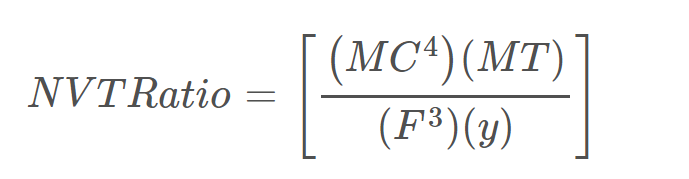## Revised (Improved) NVT Ratio Calculation for Bitcoin‘NVT’ is an acronym that stands for ‘Network Value to Transactions’ and it is a financial metric for Bitcoin that has created substantial discussion in the cryptocurrency community as of late — with many debating the usefulness (or lack thereof) of the metric itself.

Per Coinmetrics website, the definition of the NVT Ratio is,

“[A metric] ratio measures the dollar value of cryptoasset transaction activity relative to network value. This is a simple way to compare how the market prices one unit of on-chain transactions across different networks.”

In order to understand this metric, we need to dissect the different variables in the NVT ratio.

Network Value = Market Capitalization [simple as that]

We’d be remiss if we did not credit WillyWoo as the first individual to derive the concept of the NVT ratio as it applies to Bitcoin in the crypto community:

Contents

#### Woobull

Cryptocurrency Investment

## Estimated USD Transaction Value

This metric is a bit more complex to understand, but nothing too difficult.

Essentially, this metric relies on calculating the dollar value of all transactions that occurred on the network. What makes this an ‘estimation’ is that it relies on values that are not concrete and the contexts/situations surrounding each one of the transactions is unknown. Also, there are a number of exchange transactions that occur each day that are unknown.

### Interpreting This Metric

Rather than extrapolating definitions that have already been given by others in the space, we will curate our own definition for how users should interpret this metric.

Basically this metric looks at how much Bitcoin is being transacted on a daily basis (in terms of its USD value), and then its comparing that to the price.

Since that NVT line is a ratio though, the higher the ratio — the less money being spent per \$1 value of Bitcoin.

The lower the ratio, the MORE money being spent per dollar value of Bitcoin.

Therefore, if the ratio is high or increasing, that means that the price could be deterring dollar transaction activity.

That would be bearish.

However, if the ratio is low or decreasing, then that means that transactions are increasing.

# Issue #1 With the NVT Ratio in its Current Iteration

As of right now, it would appear that the NVT ratio correlates with the price, but preliminary data analysis results (running a Pearson’s coefficient) on the original NVT Ratio metric, shows that there is almost no link between the two variables.*

*Note: Regression Coefficient Obtained With Excel Spreadsheets

## Issue #2 With the NVT Ratio in its Current Iteration

The NVT Ratio is a bit incomplete.

As mentioned above, the formula for the NVT is the market capitalization for Bitcoin divided by the total Bitcoin (in USD) transacted on a given day.

The major flaw with this, however, is that whale activity could skew the price significantly.

For Example:

1. Let’s suppose that the market cap for Bitcoin today = \$10,000
2. Now, let’s suppose there were 10 transactions total today, and that the combined value of all of those transactions (in USD) was \$1,000.
3. In this instance, the NVT ratio would be 10 (10,000 to 1,000 simplified = 10:1).

But let’s switch the scenario up a little bit. Instead:

1. Let’s suppose that the market cap for Bitcoin today = \$10,000
2. Now, let’s suppose that there were 100 transactions total today, and that the combined value of all of those transactions (in USD) was \$1,000.
3. In this instance, the NVT ratio would be 10 (10,000 to 1,000 simplified = 10:1).

Clearly those two situations are not equal.

100 transactions represents way more protocol activity than just 10 transactions, but in the current NVT ratio calculation, both of them are weighed the exact same.

## Difference in the Zerononcense Renewed Calculations

Zerononcense’s calculations factor in significantly more information that the NVT ratio obtained by Willy Woo. In addition, the data is also consistent in terms of its ability to avoid hyper-sensitivity to the movements of whales.

Below is the formula derived by Zerononcense:The above formula, when ran through regression, received a score of 0.97+

Specifically, the variables are delineated as:

MC = Market Capitalization
MT = Median Transaction Value
F = USD Value of Average TX

Also, [(z*P)/(y)]=F.

So, y = total transactions (in a given 24 hour period if we’re using daily points as a plot).

# Conclusion

This financial metric will be uploaded to the website in an interactive chart version soon enough. Until then, we will continue to solicit open feedback from other researchers in the community (particularly CoinMetrics as well as WillyWoo if they have any feedback to insert).

More information and research pertaining to derived metrics will be released in the coming days and weeks from the Zerononcense platform.

This site uses Akismet to reduce spam. Learn how your comment data is processed.